Learn your way!

## Visual C# .NET 2003: Learning to Program

#### Course at a glance

Included in these subscriptions:

• Dev & IT Pro Video
• Dev & IT Pro Power Pack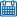Release date 5/24/2004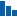Level Beginner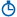Runtime 11h 3m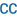Closed captioning N/A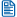Transcript N/A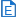eBooks / courseware Included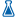Hands-on labs Included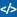Sample code Included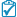Exams Included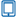Platform Internet Explorer Only##### Enterprise Solutions

Need reporting, custom learning tracks, or SCORM? Learn More

#### Course description

This course begins with an overview of C# and the .NET platform. You will learn the fundamentals of the C# language, including how to set up the application development environment, and how to write your first simple C# applications. You will learn about variables and constants, expressions and statements, operators and namespaces, and how to create classes and instantiate objects. You will be introduced to database development with ADO.NET and building ASP.NET applications. You’ll use streams to read and write data both to your local machine and across the network and the Internet. Finally, you’ll explore interoperability with legacy COM objects such as ActiveX controls and COM components.

#### Meet the expertJesse Liberty is the author of Programming C#, Programming ASP.NET, and a dozen other books on Web development and object-oriented programming. Jesse is a Distinguished Software Engineer and Architect for ATT, Ziff Davis, PBS, and Xerox. He has been a contributing author to C Report, MSDN, and other industry journals.

#### Module 1

##### Overview of .NET (07:14)
• Introduction (00:42)
• The goals (01:13)
• .NET Platform (01:03)
• .NET Features (01:11)
• .NET Resources (01:22)
• .NET Processes (01:19)
• Summary (00:23)
##### Working Examples (10:28)
• Introduction (00:32)
• Getting Started (01:33)
• Basic Windows App (01:25)
• View Event Handler (00:52)
• Language Fundamentals (01:08)
• Classes, Objects, Types (01:46)
• Methods (02:29)
• Summary (00:42)
##### Console Applications (12:48)
• Introduction (00:22)
• Comments (02:06)
• Console Objects (00:53)
• Namespaces (01:12)
• Dot Operator (00:26)
• App in Notepad (01:59)
• Compile/Run (01:18)
• Visual Studio App (03:20)
• Build/Run (00:47)
• Summary (00:24)
##### Variables and Constants (13:34)
• Introduction (00:33)
• Declarations (01:15)
• Replaceable Parameters (00:43)
• Variable Assignment (01:13)
• Constants (03:18)
• Set Variables (01:35)
• Variable Usage (02:35)
• Set Enumeration (02:01)
• Summary (00:20)
##### Programming Elements (08:29)
• Introduction (00:26)
• Types (02:39)
• Strings (00:47)
• Identifiers (00:58)
• Statement/Expression (01:35)
• Whitespace (01:35)
• Summary (00:28)
##### Branching Basics (15:23)
• Introduction (00:30)
• Branch Types (00:19)
• Unconditional (02:40)
• Basic Branching (01:08)
• Return Statements (03:38)
• Local Variables (00:40)
• Debug Branch (03:07)
• Conditional/If (02:35)
• Summary (00:44)
##### Switch/Iteration Statements (15:29)
• Introduction (00:24)
• Switch Expressions (00:39)
• String to Integer (02:49)
• Switch Statements (01:41)
• Iteration Types (02:22)
• For Statements (01:10)
• Iteration Statements (02:04)
• While/Do While (02:57)
• For Loop (00:44)
• Summary (00:38)
##### Operators (20:33)
• Introduction (00:28)
• Relational (02:20)
• Mathematical (03:12)
• Logical (03:15)
• Short Circuit Eval (02:23)
• Modulus Operator (02:20)
• Prefix/Postfix (01:52)
• Test Logicals (01:46)
• Return True/False (01:25)
• Short Circuit Test (01:11)
• Summary (00:21)

#### Module 2

##### Classes/Objects (16:33)
• Introduction (00:37)
• Access Modifiers (01:57)
• Class Methods (00:59)
• This Keyword (01:24)
• Default Constructors (00:47)
• Class Variables (01:18)
• Instantiate an Object (02:20)
• Declare an Instance (01:07)
• Add a Member Variable (01:14)
• Overload a Constructor (02:12)
• Pass in a Value (01:24)
• Review and Run (00:39)
• Summary (00:34)
##### Invoking Static Methods (08:03)
• Introduction (00:20)
• Static Members (03:55)
• Destroying Objects (01:26)
• Dispose Method (01:42)
• Summary (00:39)
##### Member Variables (19:13)
• Introduction (01:23)
• Value/Reference Types (01:49)
• Pass by Parameters (02:10)
• Pass by References (03:30)
• Pass by Values (01:19)
• Return Multiple Values (01:02)
• Out Keyword (01:28)
• Define Properties (01:01)
• Using Get and Set (03:46)
• Set a Breakpoint (01:11)
• Summary (00:33)
##### Inheritance (14:29)
• Introduction (01:37)
• Derived Classes (04:28)
• Polymorphism (03:17)
• Override Methods (01:03)
• Pass Enumerated Types (01:06)
• Class Declarations (00:55)
• Step-Through Example (01:21)
• Summary (00:41)
##### Boxing/Operator Overload (20:31)
• Introduction (01:15)
• Boxing (02:31)
• Operator Overloading (03:01)
• Type Conversion (02:52)
• Creating Type Roman (00:58)
• Computing the Value (04:00)
• Convert Integer to Roman (02:07)
• Display Results (01:08)
• Implicit Conversion (00:49)
• Explicit Conversion (01:24)
• Summary (00:24)

#### Module 3

##### Interfaces (28:15)
• Introduction (00:28)
• Implement Interface (03:38)
• Additional Classes (00:55)
• Build Interface App (00:40)
• CreateInstance (02:03)
• Create Second Interface (01:43)
• Test Second Interface (02:36)
• Interface Properties (01:57)
• Interface Methods (01:39)
• Test Extended Interface (02:07)
• Interface as a Parameter (02:04)
• Is Keyword (04:19)
• Step Through Is Code (01:21)
• As Keyword (02:20)
• Summary (00:24)
##### Arrays (10:44)
• Introduction (00:24)
• Foreach Loop (04:56)
• Create Array (01:55)
• Iterate For/Foreach (01:44)
• Initialize Array Content (01:11)
• Summary (00:34)
##### Multi-Dimensional Arrays (12:26)
• Introduction (00:36)
• Rectangular Arrays (01:45)
• Initialize Array (03:23)
• Multi-Dimensional Issues (01:39)
• Jagged Arrays (02:23)
• Params Keyword (02:11)
• Summary (00:28)
##### Indexers (12:21)
• Introduction (00:28)
• Create Indexer (04:39)
• Create/Fill Array (01:12)
• Add Offset into Array (02:26)
• String Indexer (03:16)
• Summary (00:19)
##### IEnumerable (09:56)
• Introduction (00:31)
• Implement IEnumerator (04:59)
• IEnumerated Objects (02:43)
• Step Through Enumerator (01:15)
• Summary (00:27)

#### Module 4

##### IComparables (10:55)
• Introduction (01:04)
• IComparable Interface (02:10)
• Utilizing IComparable (01:14)
• Custom Comparison (01:33)
• Custom IComparable (01:37)
• Runtime/Sort Choice (01:16)
• Creating Custom Compare (01:38)
• Summary (00:22)
##### Collection Classes (14:03)
• Introduction (00:27)
• Queues (02:13)
• Using Queue Class (02:12)
• Stacks (00:50)
• Using Stack Class (02:07)
• Stacks to Arrays (00:51)
• Dictionary/Hashtable (02:03)
• Using Hashtable (02:30)
• Summary (00:49)
##### Strings (15:29)
• Introduction (00:33)
• String Methods/Properties (02:17)
• StringBuilder Class (00:25)
• Regular Expressions (00:53)
• String Manipulations (03:11)
• Using Properties/Methods (04:01)
• Splitting with Strings (02:21)
• Splitting with Regex (01:25)
• Summary (00:23)
##### Exceptions (16:21)
• Introduction (00:44)
• Try/Catch/Finally (05:15)
• Throw an Exception (01:41)
• Using a Catch Block (02:32)
• Special Exception Handler (02:22)
• Finally Block (01:39)
• Using HelpLink/StackTrace (01:46)
• Summary (00:22)
##### Nested Exceptions (12:36)
• Introduction (00:50)
• Using Custom Exceptions (02:44)
• Looping Catch Blocks (01:54)
• Creating a Nesting (02:55)
• Step Through Nesting (03:37)
• Summary (00:35)
##### Delegates (22:55)
• Introduction (01:21)
• Delegating Methods (02:18)
• Implementing Delegates (00:42)
• Delegates as Parameters (06:03)
• Invoking Delegate Methods (06:35)
• Static Delegates (03:12)
• Delegates as Properties (02:13)
• Summary (00:30)
##### Multicast Delegates (07:33)
• Introduction (00:22)
• Using Multicast (04:33)
• Invoke Multiple Methods (02:11)
• Summary (00:26)

#### Module 5

##### Events (16:54)
• Introduction (00:43)
• Sample Event Program (00:30)
• Event Handling (02:49)
• Event Notification (01:23)
• Event Classes (01:53)
• Debug Event Program (02:01)
• Register Delegate to Event (02:04)
• Add Method/Set Index (01:09)
• EventArgs Instance (01:12)
• Fire New Event (00:57)
• Add Offset/Catch Event (01:21)
• Summary (00:47)
##### Windows Applications (16:18)
• Introduction (00:45)
• Basic Window Form (01:00)
• Windows App by Hand (05:15)
• Set Event Handler (02:17)
• Save/Compile (01:25)
• Use Visual Studio.NET (03:08)
• Modify Application (01:55)
• Summary (00:30)
##### Windows Forms (19:50)
• Introduction (00:28)
• Check Box (02:17)
• Radio Buttons (04:20)
• Output Label (04:34)
• Additional Controls (05:37)
• View Code/Run (02:02)
• Summary (00:28)
##### XML Comments (07:18)
• Introduction (00:53)
• Using XML Comments (03:06)
• XML Documentation (01:07)
• XML as HTML Files (01:52)
• Summary (00:18)
##### Relational Databases (24:57)
• Introduction (00:29)
• Database Fundamentals (03:15)
• Database Components (03:30)
• Database Structure (03:52)
• SQL Server Diagrams (01:30)
• Query Analyzer (05:07)
• Building Queries (04:40)
• Refining Information (02:05)
• Summary (00:25)

#### Module 6

##### ADO.NET Objects (19:52)
• Introduction (00:34)
• Object Model (01:07)
• Data Objects (02:59)
• Create ADO Applications (03:26)
• SqlDataAdapter (03:16)
• ListBox (03:39)
• OleDbDataAdapter (04:03)
• Summary (00:44)
##### Data Relationships (25:26)
• Introduction (00:37)
• DataGrids in ADO (04:09)
• SqlConnection Object (02:42)
• SQLCommand Object (00:55)
• DataAdapter (02:02)
• Populate DataGrid (01:45)
• Relating Data (04:53)
• DataRelation Object (03:57)
• Test/Debug ADO App (00:26)
• Use Debug Tools (02:34)
• Show Order Details (00:59)
• Summary (00:22)
##### Updates (16:03)
• Introduction (00:28)
• Populate ListBox (04:29)
• Update Displayed Data (02:14)
• Update Drop-Down Menu (01:39)
• Update Database Data (04:21)
• Update Multiple Tables (02:32)
• Summary (00:16)
##### Transactions (14:36)
• Introduction (00:53)
• Stored Procedures (03:47)
• Use Stored Procedures (02:07)
• Pass Parameters (02:42)
• Transaction Support (00:59)
• Connection Transaction (01:06)
• Commit/Rollback (02:25)
• Summary (00:34)
##### Update Using DataAdapter (11:07)
• Introduction (00:36)
• DataSet Method (02:51)
• Map Column Names (02:08)
• Update with ADO.NET (03:17)
• Update with DataAdapter (01:47)
• Summary (00:26)

#### Module 7

##### Concurrency (11:48)
• Introduction (00:46)
• Sproc (01:39)
• Params for Concurrency (02:00)
• ADO.NET w/Concurrency (03:43)
• CommandBuilder (03:09)
• Summary (00:28)
##### Web Forms (28:13)
• Introduction (00:55)
• ASP.NET Web App (05:38)
• Add Label/Button (01:41)
• Run Web App (00:58)
• Controls (01:51)
• ASP.NET Events (01:51)
• DataGrid Controls (03:00)
• Bind DataGrid (03:22)
• Event Handling TableCells (00:57)
• Create New Method (02:52)
• Create DataRowView (00:34)
• Extract DataItem (03:59)
• Summary (00:30)
##### Attributes (10:18)
• Introduction (00:43)
• Attribute Targets (00:50)
• Custom Attributes (01:10)
• Create Custom Attributes (00:29)
• Use Attributes (03:54)
• Named Attributes (02:00)
• ILDasm (00:54)
• Summary (00:16)
##### Reflections (06:47)
• Introduction (00:43)
• Array of Attributes (04:00)
• Discovery (01:33)
• Summary (00:29)

#### Module 8

##### Streaming Data (13:27)
• Introduction (00:36)
• Explore Directory (04:25)
• Read/Write Streams (03:39)
• Buffered Stream (02:12)
• StreamReader/Writer (02:07)
• Summary (00:27)
##### Asynchronous I/O (08:18)
• Introduction (00:39)
• Callback Delegate (00:31)
• Test Constructor (00:10)
• Buffers/Token (00:53)
• Buffering at Work (00:23)
• HttpWebRequest (03:28)
• Read Page from Web (00:18)
• Web Streaming Objects (00:59)
• Summary (00:53)
##### Network I/O (26:00)
• Introduction (00:27)
• Network I/O (00:24)
• Sockets/Ports (00:17)
• Support Network I/O (02:22)
• Server Side (03:53)
• Client Side (02:15)
• Server/Client Interaction (01:59)
• Client Handler (02:29)
• Asynch Read/Write (04:02)
• Send Text String (02:12)
• Asynch File Read (03:08)
• Asynch File Streaming (02:09)
• Summary (00:16)
##### Serialization (10:20)
• Introduction (00:30)
• Binary Serialization (01:15)
• Serialize to Disk (02:57)
• Deserialize (01:58)
• Nonserialized Option (03:21)
• Summary (00:17)
##### ActiveX (19:17)
• Introduction (00:29)
• Create ActiveX Control (00:32)
• Create VB Project (06:30)
• ActiveX Event Handler (03:02)
• Create .NET Project (00:06)
• Register Control (01:34)
• Add Control to Toolbox (05:53)
• Summary (01:08)